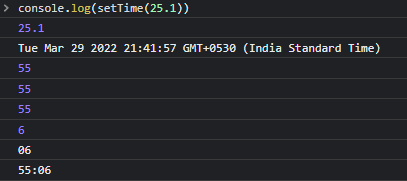# 25 + 5 Clock issue with b.apply is not a function

I’m doing the 25 + 5 clock challenge to claim the Front-end Libraries certification, I’m having problems understanding why is failing and gives a ‘b.apply is not a function’ if you check the link that I shared to codepen and check the the clock is working as intended, but the some of tests fails with this error and I other is that isn’t showing the value when is counting, I’ll appreciate any help to understand why is failing and any indication of how I can fix it, thanks.

`````` setTime(time) {
let number = new Date();

let a = number.setMinutes(time)
console.log(time)
let minutes = Math.floor(a % (1000 * 60 * 60) / (1000 * 60));
console.log(minutes)
minutes = minutes < 10 ? "0" + minutes : minutes;
minutes = time * 60 > 3599 ? time : minutes;

number.setSeconds(time * 60);
let seconds = Math.floor(number % (1000 * 60) / 1000);
seconds = seconds < 10 ? "0" + seconds : seconds;

return minutes + ':' + seconds;
}
``````

Take a look at this function. You are feeding 25 as argument to this function and its returning “55:00” instead of “25:00”

Hi thanks for answering, I checked/looked the code and don’t see where’s returning 55, here’s a screenshot.

I used quokka on vscode to show the output

The code

``````const setTime = (time) => {
let number = new Date();
console.log(time);
console.log(number);

let minutes = Math.floor(number.setMinutes(time) % (1000 * 60 * 60) / (1000 * 60));
console.log(minutes);

minutes = minutes < 10 ? "0" + minutes : minutes;
console.log(minutes);

minutes = time * 60 > 3599 ? time : minutes;
console.log(minutes);

let seconds = Math.floor(number.setSeconds(time * 60) % (1000 * 60) / 1000);
console.log(seconds);

seconds = seconds < 10 ? "0" + seconds : seconds;
console.log(seconds);

return minutes + ':' + seconds;
}

console.log(setTime(25.1));
``````

call setTime(25) and checkI think its somehow depends on the time region

This topic was automatically closed 182 days after the last reply. New replies are no longer allowed.